# Fraction To Decimal Converter

Created by Rita Rain
Reviewed by Dominik Czernia, PhD candidate and Jack Bowater
Last updated: Jul 14, 2020

If "how to turn a fraction into a decimal" has left you in a pickle recently, you've found the right tool - this fraction to decimal converter. It is fast, easy-to-use, and even lets you set the rounding. In the text, you'll find out how to use this fraction to decimal calculator, what numerators and denominators are, what is 7/8 as a decimal, and how to convert a fraction to a decimal. In case you'd like to perform the reverse operation, we've also made a decimal to fraction calculator. You can convert fractions to percents too.

## How to use the fraction to decimal converter

1. Choose the fraction form. If the fraction doesn't include a whole number, leave "simple," if it does - choose "mixed."

2. Input the top part of your fraction into the "numerator" field, and the bottom part into the "denominator" field of the fraction to decimal calculator. Input the whole number too, if you've chosen the mixed number option.

3. You can set to how many decimal places you want to round the result to in the fourth field of the fraction to decimal converter. If you choose the custom option, another field will pop up in the fraction to decimal calculator. You can round the decimal up to 15 places.

4. The calculator will convert the fraction to a decimal and print the result at the bottom.

## Fractions and decimals - introduction

Decimals and fractions are ways of representing numbers. Fractions are those numbers in the "n/d" form. The top part of the fraction (n) is called the numerator, and the bottom part (d) is the denominator.

Decimals are represented as "a.b" where a is the whole number, and b is the decimal part. Below you can see decimals on the left and their equivalent fractions on the right:

0.1 = 1/10

0.01 = 1/100

0.001 = 1/1000

(...)

Analogously:

0.45 = 45/100

0.623 = 623/1000

Note that the number of digits after the decimal point is equal to the number of zeros in the denominator of the fraction.

## How to convert a fraction to a decimal by hand - the first method

1. If it's possible, find the equivalent fraction with a power of 10 as the denominator.
• You can do that by multiplying or dividing both the numerator and denominator of the original form by the same number. The operation doesn't change the value, because you are, in effect, multiplying by 1 (2/2 in the example below).

• Do this in a way that eventually makes the numerator a whole number. For example: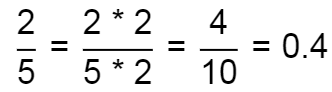1. Then change the form to decimal. For example:

4/10 = 0.4

## How to turn a fraction into a decimal by hand - the second method

You can also convert a fraction into a decimal by performing a division:

1/4 = 1:4

You can type this into a calculator or do the long division: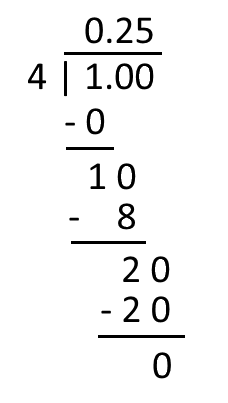## 7/8 as a decimal - fraction to decimal examples

Let's have a look at a few examples of how to turn a fraction into a decimal: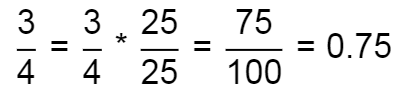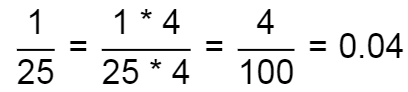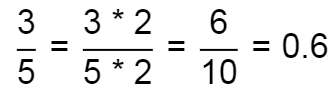For 7/8, do long division: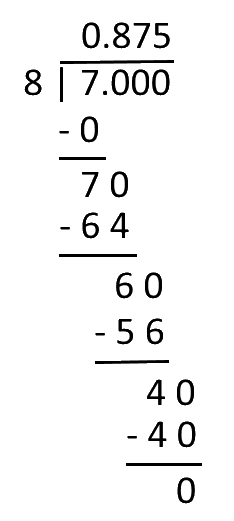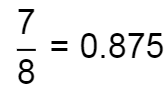Rita RainFraction form
Simple
Numerator (n)
Denominator (d)
Round to
10 decimal places
Result
People also viewed…

### Eggs

Quantum physicist's take on boiling a perfect egg.

### Mixed number to improper fraction

This mixed number to improper fraction calculator works like a charm - it not only converts one fraction form to the other, but also it shows the step by step solution.

### Perfect square trinomial

Perfect square trinomial calculator checks if your trinomial is a perfect square of a linear binomial and, if so, finds the binomial.

### Sunbathing

Do you always remember to put on sunscreen before going outside? Are you sure that you use enough? The Sunbathing Calculator ☀ will tell you when's the time to go back under an umbrella not to suffer from a sunburn!